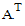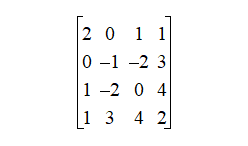# NMath User's Guide

17.3 Symmetric Matrices (.NET, C#, CSharp, VB, Visual Basic, F#)

A symmetric matrix is a square matrix that satisfieswheredenotes the transpose of. That is,for all. For example, this is a 4 x 4 symmetric matrix:Symmetric matrices are often used to represent quadratic forms.

NMath provides symmetric matrix classes for single- and double-precision floating point numbers. The classnames are and . Hermitian matrices are a generalization of symmetric matrices for complex types (Section 17.4).

For efficiency, only the upper triangle is stored. The storage scheme is the same as for an upper triangular matrix (Section 17.2).

Top

Top